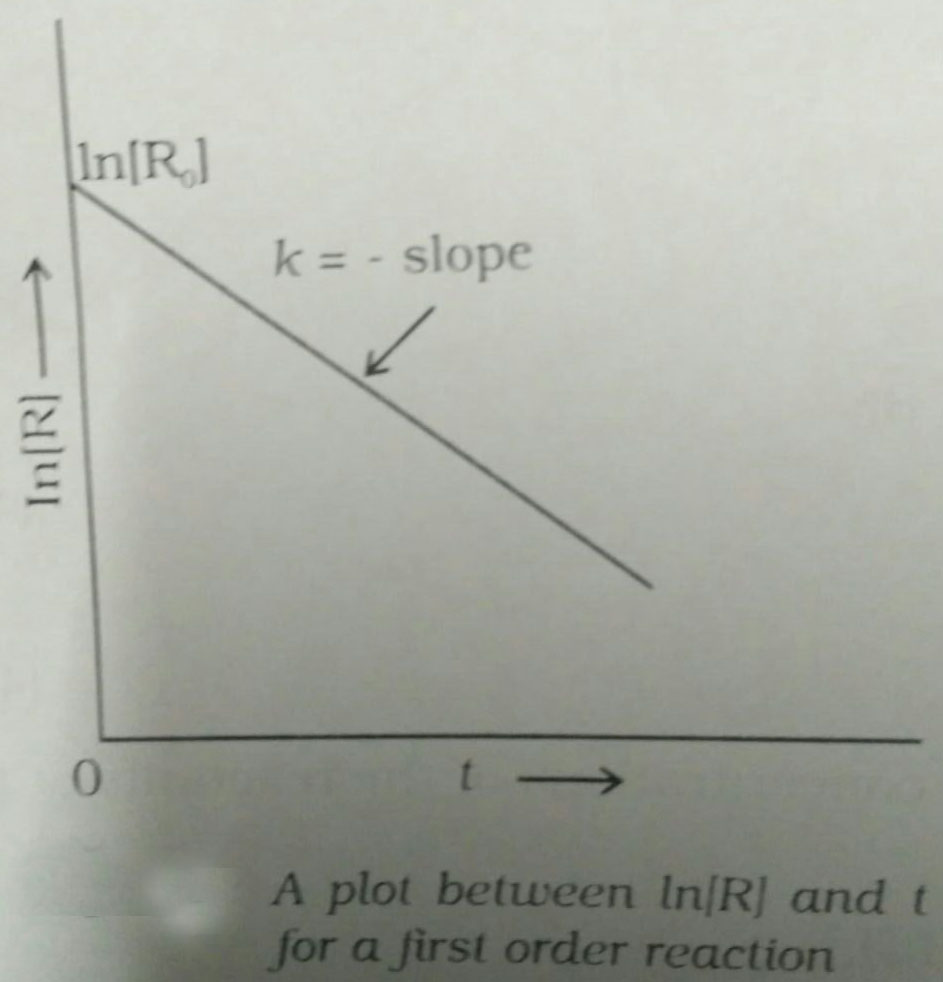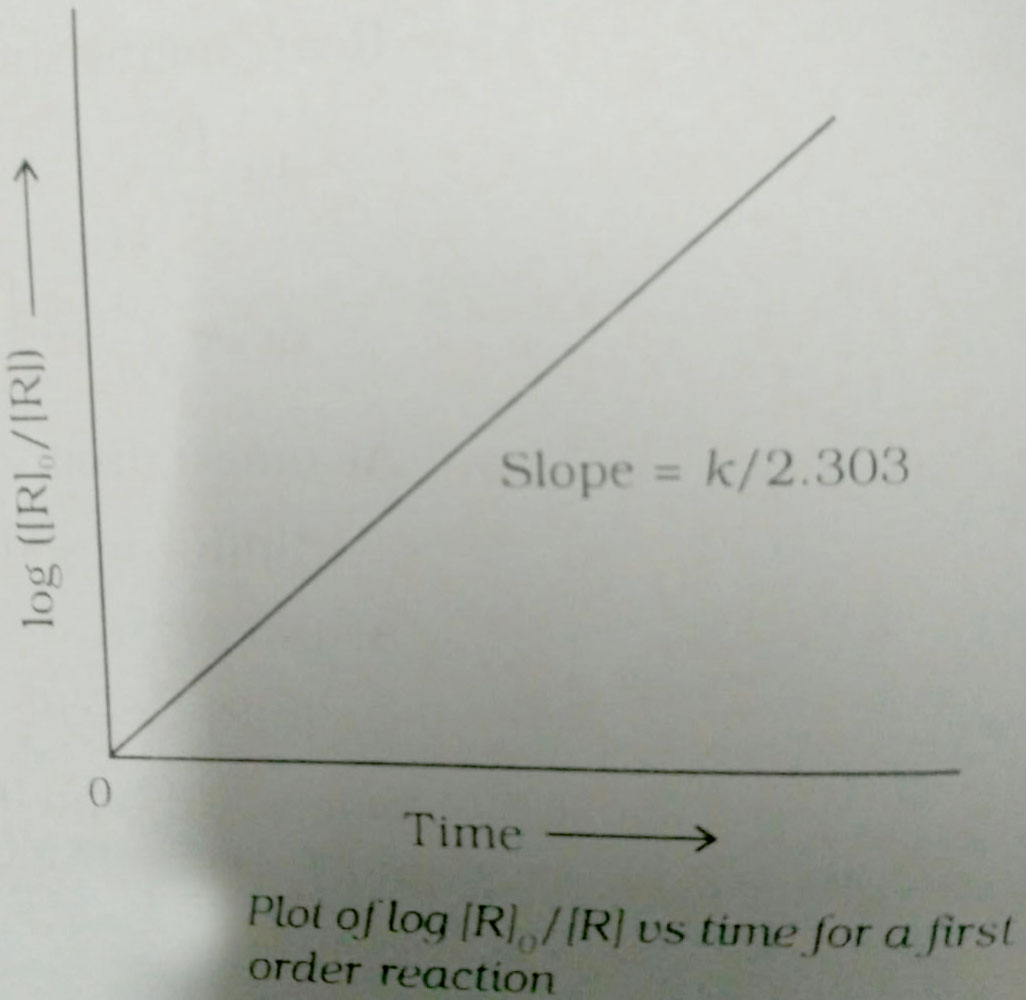#### A graph plotted betweenvs loga concn is a straight line. What conclusion can you drow from the given graph?(n=order) Option 1)Option 2)Option 3)Option 4) noneAs we leaned in concept

First Order Reaction -

The rate of the reaction is proportional to the first power of the concentration of the reaction- wherein

Formula:

RP

a                 0

a-x             x[differentiate rate law]Unit ofWe know that for first order reactions,So, in other words, in a first oder reaction,does not depend on the concentration.

Therefore, graphically we can draw.

n=1,Option 1)Incorrect option

Option 2)Incorrect option

Option 3)Correct option

Option 4)

none

Incorrect option